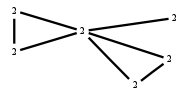# The Markov Bases Database

## G117g_bin

The binary graphical model of G117.

It is a hierarchical model of 6 variables. The dimension of the model is 15.The cardinality of the statespace is 64.

### Properties of the Markov basis

 Markov degree 2 456

 degree 2 456

The model has the following properties:

• All variables are binary.
• It is a graphical model.
• The semigroup is normal.### FILES

 Markov basis: sufficient statistics matrix: G117g_bin.mar (85.95 kb) G117g_bin.mat (2.53 kb) G117g_bin.mod (39 b) G117g_bin.tar.gz (8.43 kb)